# Team:Duke/Modeling/2

•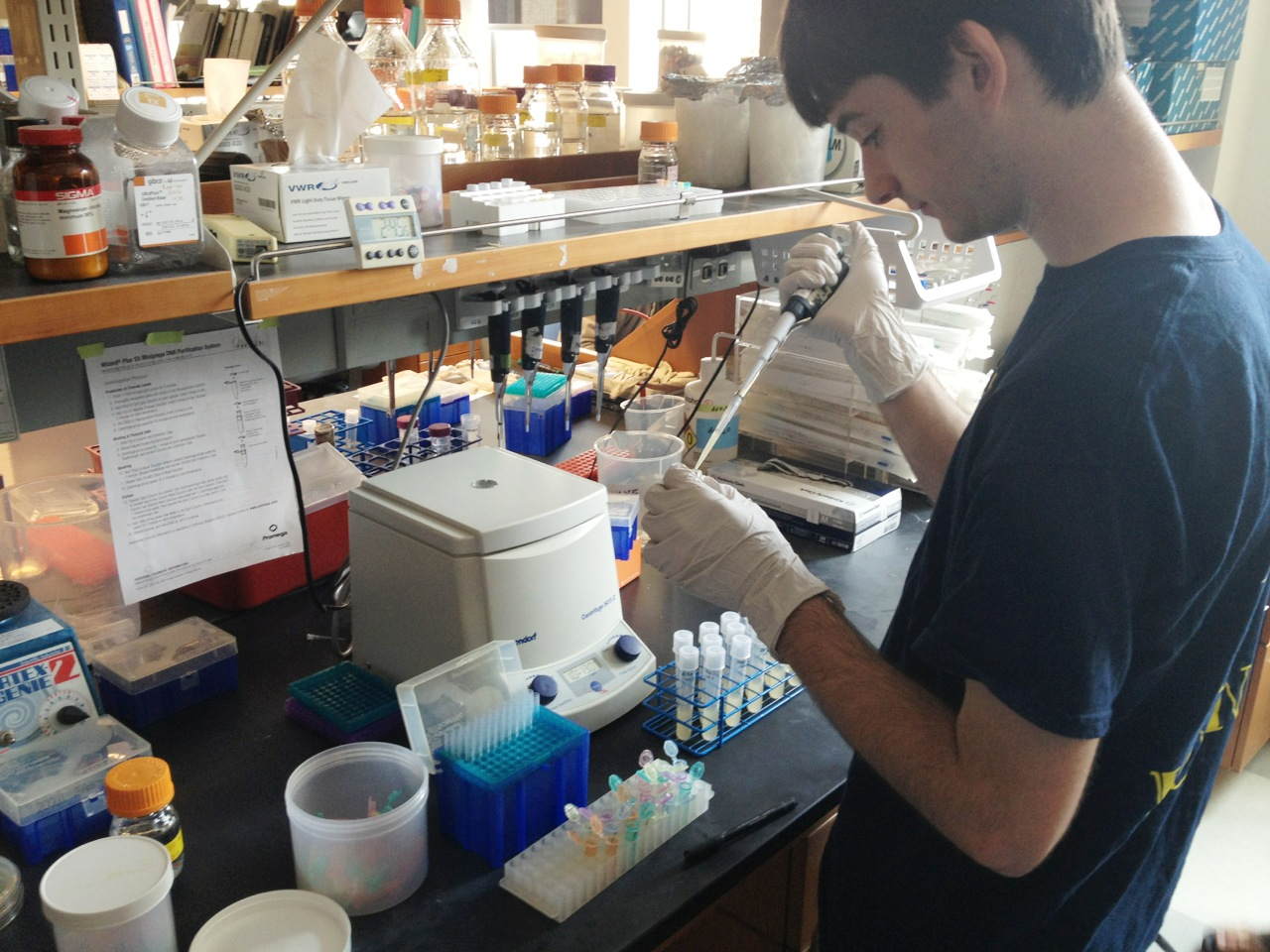•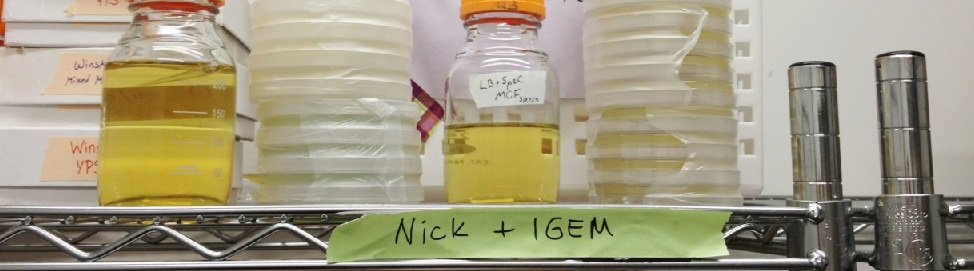•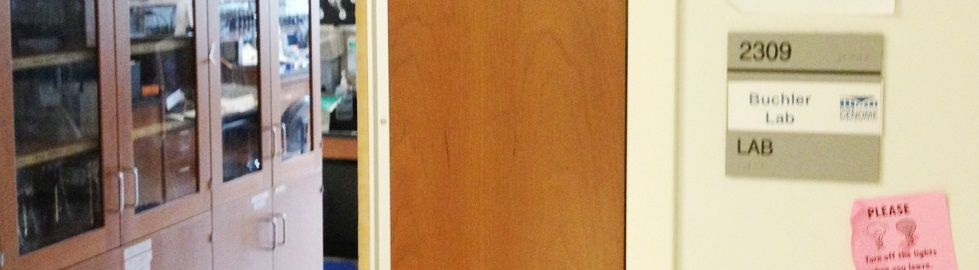•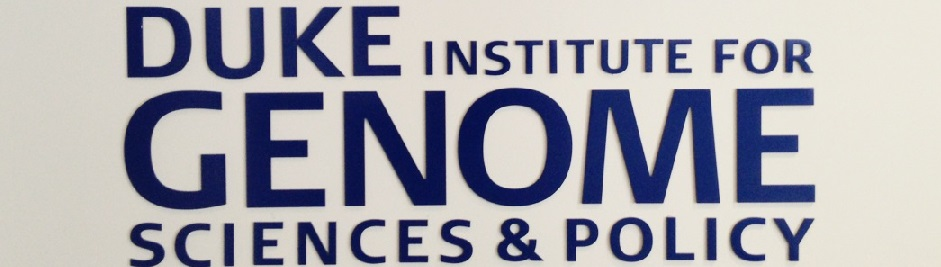•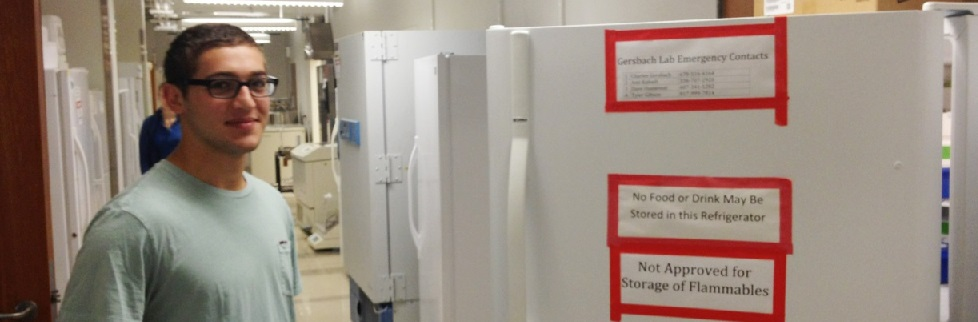•••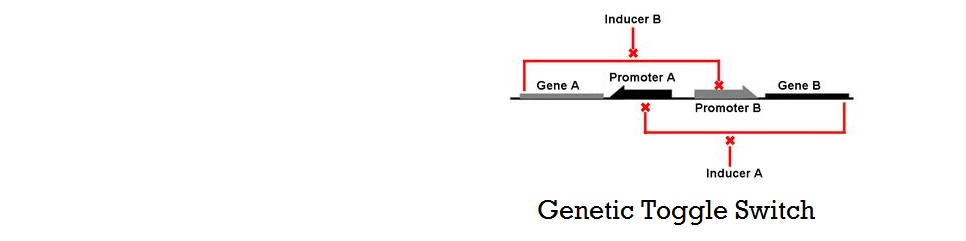•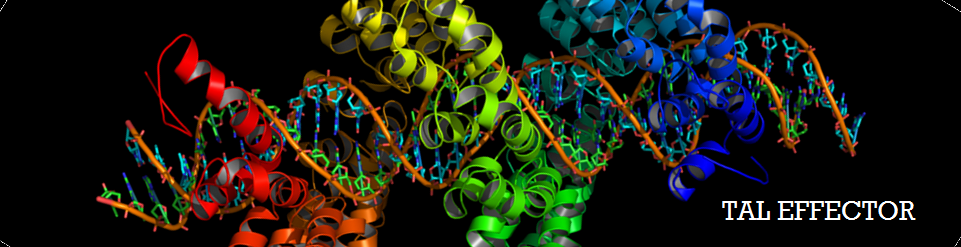•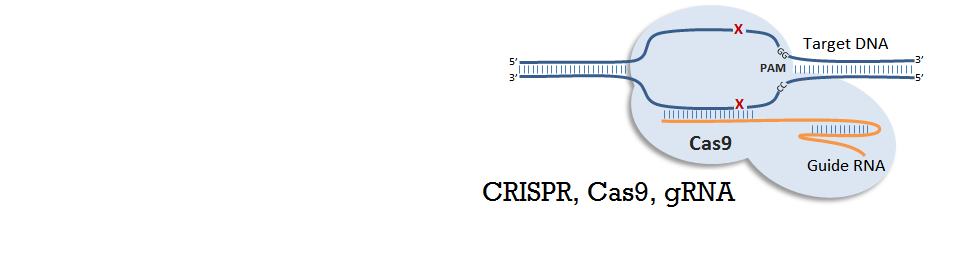# Mathematical Modeling of Bistable Toggle Switch

## Introduction to Thermodynamic Model of Cooperative Repression

A thermodynamic approach was used to model the cooperative repression by transcription factors (iTAL and CRISPR) binding to multiple binding sites. The model was largely based on the models shown in "Transcriptional regulation by the numbers: models" (Bintu, 2005), which base on the reasoning that gene expression level is related to the probabilities of various molecules--such as transcription factors (TFs) and RNA Polymerase (RNAP)--binding to the DNA of interest. The key concept in this model is using the "probability that RNAP occupies the promoter of interest" instead of using "concentration of protein" produced. One of the most important assumptions of this model is that promoter occupancy by RNAP is directly proportional to the level of gene expression.

Figure 1. Key Assumption of the Thermodynamic Model

### Simple Case (No Transcription Factors)

First, let's start with the case without any transcription factors. In this simple case, we will ignore the RNAPs that are in the cytoplasm or engaged in transcription, and assume that our pool of free RNAPs is the "non-specifically" bound RNAPs (Non-specific here means the protein is bound to sequences other than the target promoter). Then, the sum of Boltzmann weights of all possible states of P polymerase molecules on DNA must be calculated to evaluate the probability of RNAP bound. Shown below is a table of variables used in the calculations that follow.

Figure 2. Table of Variables

All the possible outcome of P RNAP molecules binding to the genome can be classified into two:

1. all RNAP bound non-specifically, or
2. (P-1) RNAP bound non-specifically and 1 RNAP bound specifically to promoter of interest.

Next, we count the number of ways these two outcomes can be achieved and sum their statistical weights calculated by:

Figure 3. Statistical Weight (Boltzmann Weight)

Using the above formula and combinatorics, the statistical weight of having P RNAP distributed among Nns non-specific sites can be calculated by the following:

Figure 4. Statistical Weight of State with Unoccupied Promoter

Similarly, the statistical weight of having (P-1) RNAP bound non-specifically and one RNAP bound specifically to the promoter of interest can be calculated by:

Figure 5. Statistical Weight of State with Occupied Promoter

To obtain the probability of RNAP bound to promoter of interest, we simply divide the statistical weight of specific-binding by the total statistical weight of both states. When simplified, the following equation for probability of RNAP bound to promoter is found. (Detailed derivation can be found here: Derivation)

Figure 6. Equation for Probability of RNAP Bound

### Case with Transcription Factors

When activators and repressors are present, similar derivation using boltzmann weights of all different states can be used to derive the equations for probability of RNAP bound. For example, having an activator will lead to four different states (P: RNAP, A: Activator):

1. Z(P,A) : all non-specific binding
2. Z(P-1,A) : RNAP bound to promoter specifically
3. Z(P,A-1) : activator bound specifically
4. Z(P-1,A-1) : both RNAP and activator bound

Likewise, the presence of repressors give rise to three states (P: RNAP, A: Repressor). Notice there is no state Z(P-1,R-1) with both RNAP and repressor bound.

1. Z(P,R) : all non-specific binding
2. Z(P-1,R) : RNAP bound to promoter specifically
3. Z(P,R-1) : repressor bound specifically

For the case of repressors, probability of RNAP bound can be found by the same method: taking the ratio of Boltzmann weights. After simplifying, it turns out that the having repressors introduces a new term, called F-reg (regulation factor). A step-by-step derivation of the equation can be found here: Derivation).

Figure 7. Probability of RNAP Bound in the Presence of Repressors

Repressors will have F-reg values that are less than 0, and such values increase the denominator in the equation for probability of RNAP bound. Thus, repressor lowers the probability of RNAP bound. In addition, our experimental design, which consists of multiple binding sites for the same repressor, falls under the category of "non-overlapping activators and/or repressors acting independently on RNAP". When transcription factors affect RNAP independently, the total F-reg equals to the product of all F-regs, or equivalently,

Figure 8. Total Regulation Factor for Multiple TFs

For a additional explanation on this thermodynamic model, refer to the publication: Bintu, et al. 2005) With this understanding of the basic assumptions, derivations, and meaning of the thermodynamic model, we continue to model the cooperative repression via multiple binding sites in the next page).